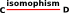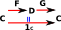# Maths - Isomorphism

 An isomorphism between two mathematical objects is a comparison between the two objects similar to equality. However an isomorphism is weaker and more general.• Two objects are equal if they are exactly the same in every respect.
• Two objects are isomorphic if they have exactly the same mathematical properties.

For sets and mathematical structures based on sets we can define isomorphism using mappings in the following precise way:

 Given two mathematical objects 'C' and 'D' that we want to compare, we construct two morphisms between them 'F' and 'G' as shown in this diagram:'F' and 'G' must be chosen such that, by applying them one after the other (composing them), we get back exactly where we started, That is: GF = 1CFG = 1DIf we can do this then the objects 'C' and 'D' are isomorphic to each other.

Isomorphism is weaker than equality but it is defined using equality of mappings. That is, by using equality of mappings we can only compare the objects 'upto isomorphism'.

### Isomorphism in Category Theory

We have seen that the concept of isomorphism does not need to be defined by comparing the internal structures: the isomorphism is defined purely in terms of the external mapping of the structures. This idea of defining structures externally is one of the key characteristics of a branch of mathematics called category theory as will be discussed on this page.

Category theory gives us a way, not just to compare structures by equality and isomorphism, but others such as equivalence and adjunction. This turns out to be very powerful.

Where I can, I have put links to Amazon for books that are relevant to the subject, click on the appropriate country flag to get more details of the book or to buy it from them.Contemporary Abstract Algebra written by Joseph Gallian - This investigates algebra (Groups, Rings, Fields) using an abstract (set theory) approach. It is very readable for such an abstract topic but the inclusion of 1 page histories of the great mathematicians and other background information.